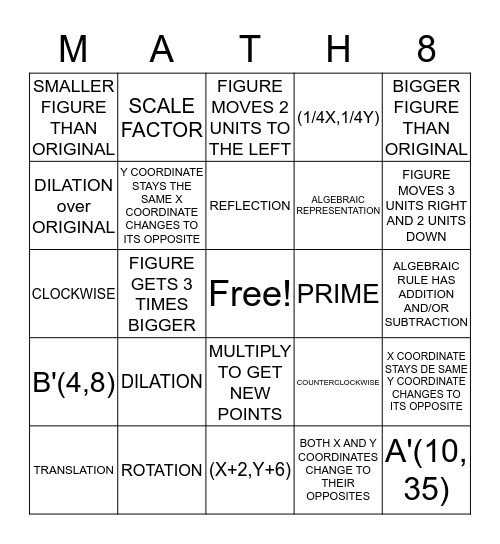# TransformationsThis bingo card has a free space and 24 words: DILATION, ROTATION, REFLECTION, TRANSLATION, BIGGER FIGURE THAN ORIGINAL, SMALLER FIGURE THAN ORIGINAL, DILATION over ORIGINAL, SCALE FACTOR, PRIME, MULTIPLY TO GET NEW POINTS, ALGEBRAIC REPRESENTATION, CLOCKWISE, COUNTERCLOCKWISE, X COORDINATE STAYS DE SAME Y COORDINATE CHANGES TO ITS OPPOSITE, Y COORDINATE STAYS THE SAME X COORDINATE CHANGES TO ITS OPPOSITE, BOTH X AND Y COORDINATES CHANGE TO THEIR OPPOSITES, ALGEBRAIC RULE HAS ADDITION AND/OR SUBTRACTION, FIGURE MOVES 2 UNITS TO THE LEFT, FIGURE MOVES 3 UNITS RIGHT AND 2 UNITS DOWN, FIGURE GETS 3 TIMES BIGGER, A'(10, 35), B'(4,8), (1/4X,1/4Y) and (X+2,Y+6).

## Play Online